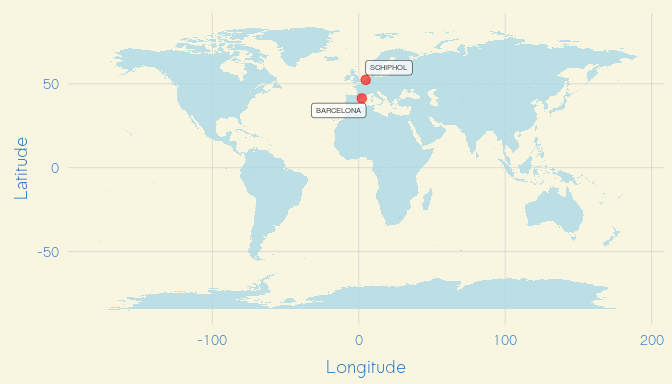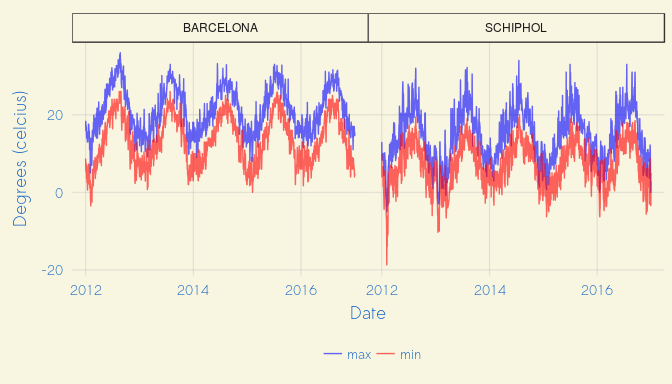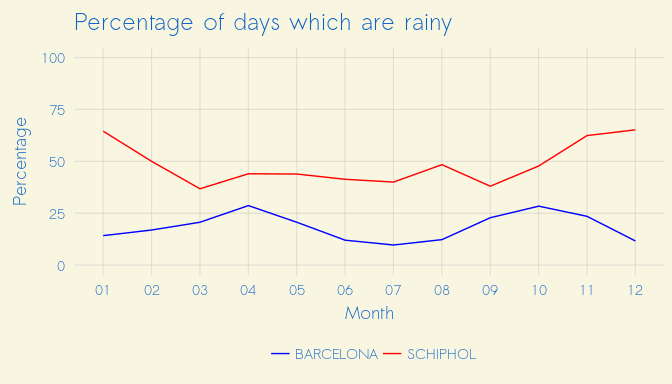# gsod: Utilities for exploring NOAA GSOD weather data

#### 2017-12-13

gsod, the weather package of DataBrew, is an effort to streamline the way that researchers and data scientists interact with and utilize weather data.

## Objectives

The objectives of gsod package are simple:

1. Make accessing historical weather data easy.

2. Make accessing historical weather data fast.

## Installation

To use the gsod package, you’ll first need to install it. With a good internet connection, run the following from within R.

if(!require(devtools)) install.packages("devtools")
install_github('databrew/gsod')

Once you’ve installed the package, you can use its functionality in any R script by simply including the following line:

library(gsod)

## Basic use

Let’s settle the debate once and for all: which has better weather, the Dutch capital of Amsterdam or the Catalan capital of Barcelona.

# Get weather for 2012-2016
library(gsod)
weather <- bind_rows(gsod2012,
gsod2013,
gsod2014,
gsod2015,
gsod2016)

Now we’ll find the nearest weather stations to our locations of interest through the gsod package’s find_nearest_station function:

# Get the station ids
ids <- bind_rows(find_nearest_station('Amsterdam, Netherlands'),
find_nearest_station('Barcelona, Catalonia'))
ids
         stnid   lon    lat       km  stn_name
1 062400-99999 4.764 52.309 11.65975  SCHIPHOL
2 081810-99999 2.078 41.297 13.80614 BARCELONA

Next, we’ll plot our weather stations on a world map:

library(rworldmap)
library(ggplot2)
library(ggrepel)
library(databrew)
world <- map_data(map="world")

g <-
ggplot() +
theme_databrew() +
geom_map(data=world,
map=world,
aes(map_id=region, x=long, y=lat),
fill = 'lightblue',
alpha = 0.8) +
geom_point(data = ids,
aes(x = lon,
y = lat),
alpha = 0.6,
size = 3,
color = 'red') +
labs(x = 'Longitude',
y = 'Latitude') +
theme(legend.position = 'bottom') +
geom_label_repel(data = ids,
aes(x = lon,
y = lat,
label = stn_name),
alpha = 0.7,
size = 2)
gTo start, we’ll have a look at the daily high and low temperatures.

# Get weather for only our two stations
weather <- weather %>%
filter(stnid %in% ids\$stnid)
# Make long tidy data for plotting temperatures
long <- weather %>%
dplyr::select(date, max, min, stn_name) %>%
gather(key, value, max:min)
# Compare temperature
g <- ggplot(data = long,
aes(x = date,
y = value,
color = key)) +
geom_line(alpha = 0.6) +
facet_wrap(~stn_name) +
theme(legend.position = 'bottom') +
scale_color_manual(name = '',
values = c('blue', 'red')) +
theme_databrew() +
labs(x = 'Date',
y = 'Degrees (celcius)')
gFor many, rainfall matters more than temperature. Let’s have a look at the percentage of days per month which have any precipitation:

rain <- weather %>%
mutate(month = format(date, '%m')) %>%
group_by(month, stn_name) %>%
summarise(rainy = length(which(prcp > 0)),
days = n()) %>%
ungroup %>%
mutate(p = rainy / days * 100)

ggplot(data = rain,
aes(x = month,
y = p,
color = stn_name,
group = stn_name)) +
geom_line() +
theme_databrew() +
scale_color_manual(name = '',
values = c('blue', 'red')) +
labs(x = 'Month',
y = 'Percentage',
title = 'Percentage of days which are rainy') +
ylim(0,100)## Conclusion

Amsterdam is great if you like the cold and the rain.

## Details

Head over to the gsod package page.